# Impulse and momentum

## (3) Impulse and Linear momentum

• To explain the terms impulse and momentum consider a particle of mass m is moving along x-axis under the action of constant force F as shown below in the figure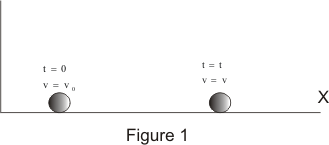• If at time t=0 ,velocity of the particle is v0 then at any time t velocity of particle is given by the equation
v = v0 + at
where a = F/m
can be determined from the newton's second law of motion .Putting value of acceleration in above equation\
we get
mv = mv0 + Ft
or
Ft = mv - mv0                   -(1)
• right side of the equation Ft, is the product of force and the time during which the force acts and is known as the impulse
Thus
Impulse= Ft
• If a constant force acts on a body during a time from t1 and t2,then impulse of the force is
I = F(t2-t1)                  -(2)
Thus impulse received during an impact is defined as the product of the force and time interval during which it acts
• Again consider left hand side of the equation (1) which is the difference of the product of mass and velocity of the particle at two different times t=0 and t=t
• This product of mass and velocity is known as linear momentum and is represented by the symbol p. Mathematically
p = mv                  --(3)
• physically equation (1) states that the impulse of force from time t=0 to t=t is equal to the change in linear momentum during

• If at time t1 velocity of the particle is v1 and at time t2 velocity of the particle is v2,then
F(t2-t1)=mv2-mv1                  -(4)
• so far we have considered the case of the particle moving in a straight line i.e along x-axis and quantities involved F,v, and a were all scalars
• If we call these quantities as components of the vectors F,v and a along x-axis and generalize the definitions of momentum and impulse so that the motion now is not constrained along one -direction ,Thus we got
Impulse=I=F(t2-t1)                  -(5)
Linear momentum=p=mv                  -(6)
where
I=Ixi+Iyj+Izk
F=Fxi+Fyj+Fzk
p=pxi+pyj+pzk
v=vxi+vyj+vzk
are expressed in terms of their components along x,y and z axis and also in terms of unit vectors
• On generalizing equation (4) using respective vectors quantities we get the equation
F(t2-t1) =mv2-mv1                  -(7)
• So far while discussing Impulse and momentum we have considered force acting on particle is constant in direction and magnitude
• In general ,the magnitude of the force may vary with time or both the direction and magnitude may vary with time
• Consider a particle of mass m moving in a three-dimensional space and is acted upon by the varying resultant force F. Now from newtons second law of motion we know that
F=m(dv/dt)
or Fdt=mdv
• If at time t1 velocity of the particle is v1 and at time t2 velocity of the particle is v2,then from above equation we have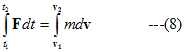• Integral on the left hand side of the equation (8) is the impulse of the force F in the time interval (t2-t1) and is a vector quantity,Thus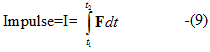Above integral can be calculated easily if the Force F is some known function of time t i.e.,
F=F(t)

• Integral on the right side is when evaluated gives the product of the mass of the particle and change in the velocity of the particle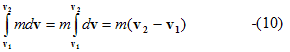• using equation (9) and (10) to rewrite the equation (8) we get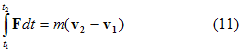• Equivalent equations of equation (11) for particle moving in space are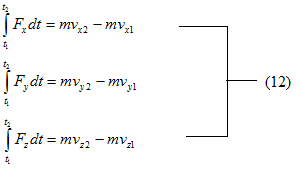• Thus we conclude that impulse of force F during the time interval t2-t1 is equal to the change in the linear momentum of the body on which its acts

• SI units of impulse is Ns or Kgms-1
Example 1
A batsman hits back a ball straight in the direction of the bowler without changing its initial speed of 5 m/s If the mass of the ball is 0.20 kg, determine the impulse imparted to the ball. (Assume linear motion of the ball)
Solution
Impulse =change in momentum
=.20 * 5 - (-.20* 5)
=2 N s
Watch this tutorial for more information on How to solve momentum/Impulse problems